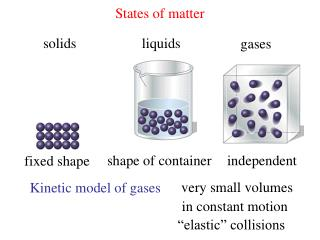DownloadDownload PresentationStates of matter

# States of matter

Télécharger la présentation## States of matter

- - - - - - - - - - - - - - - - - - - - - - - - - - - E N D - - - - - - - - - - - - - - - - - - - - - - - - - - -
##### Presentation Transcript

1. States of matter solids liquids gases shape of container independent fixed shape very small volumes Kinetic model of gases in constant motion “elastic” collisions

2. Pressure = force force = collisions of particles area 14.7 lbs sea level atmospheric pressure ft2 vacuum = absence of gas particles

3. barometer atmospheric pressure Torricelli 1 atm 760 mm Hg = 760 torr 760 mm Hg = 1.01325 x 105 Pa 760 mm Hg = could use water 10,300 mm H2O 34 feet

4. Boyle’s Law Pressure Volume PV = constant

5. Boyle’s Law Pressure Volume PV = constant = P2V2 P1V1 A sample of Cl2 has a volume of 946 mL at a pressure of 726 mm Hg. If the volume is reduced to 154 mL, calculate the pressure. (726 mm Hg) (946 mL) = P2 (154 mL) P2 = 4460 mm Hg  760 mm Hg/atm = 5.97 atm 4460 mm Hg

6. Charles’s Law Volume Temperature V = constant T Kelvin V = 0 T = -273.15oC

7. V1 V2 T1 T2 Charles’s Law Volume Temperature = 273.15 K A container is filled with N2 in an ice bath (0o C) Its volume is 58.7 mL. It is then put in a bath of boiling isopropanol. Its volume goes to 76.4 mL. What is the b.p. of isopropanol? 76.4 mL T2 = 58.7 mL 355.5 K = 273.15 K 82.3o C T2

8. Avogadro’s Law Volume moles Gay-Lussac  2 H2 + O2 2 H2O (g) Law of combining volumes 200 mL H2 100 mL O2 200 mL H2O Avogadro equal volumes of gases = equal numbers of molecules 1 mol gas = 6.023 x 1023 particles = 22.4 L at standard Temperature and Pressure 0.0oC (273.2 K) 1 atm (760 torr)

9. Ideal Gas Law Boyle PV = constant P V = n R T Charles R V = ideal gas constant = constant T = 0.08206 L atm V Avogadro = constant mol K n two types of problems 1. Predicting properties of a system PV = nRT no changes 2. Changing conditions P1V1 = R = P2V2 n1T1 n2T2

10. Calculate the pressure of 80.10 g. of CO2 gas in a 5000 mLflask at 70.0oC 1. Predicting properties of a system PV = nRT P = V = 5000 mL = 5.00 L n = 80.10 g ÷ 44.01 g/mol = 1.82 mol T = 70.2 + 273.15 = 343.2 K (P) (5.00 L) = (1.82 mol) (0.08206 L atm) ( 343.2 K) mol K (P) = 10.25 atm

11. P2V2 P1V1 n2T2 n1T1 A small bubble (2.1 mL) rises from the bottom of a lake, where the temperature is 8.00C and the pressure is 6.4 atm, to the surface where the temperature is 25.0oC. What is the volume of the bubble at the surface? initial final 6.4 atm 1.0 atm P1= P2= V1 = V2 = 14 mL 2.1 x 10-3 L T1 = 281.15 K T2 = 298.15 K 2. Changing conditions = R =